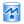TheSurface Current Sensor Definition allows users to collect either transient or steady-state data. A definition must accompany a surface current sensor in order to specify where the data will be collected. The equations for transient and steady-state surface currents are

\begin{eqnarray} J_s(t) &=& \hat n\times H(t) \\ \tilde {J_s} &=& \hat n\times \tilde H \end{eqnarray}

where

$J_s(t) =$ time-domain surface current

$H(t) =$ time-domain magnetic field tangent to the outside of the surface

$\tilde {J_s} =$ frequency-domain complex surface current phasor

$\tilde H =$ frequency-domain complex magnetic field phasor tangent to the outside of the surface

$\hat n =$ surface normal

Users can access the Surface Current Sensor Definition Editor by either right-clicking on the Definitions branch of the Project Tree and selecting New Surface Current Sensor Definition, or double-clicking on an existing definition in the Project Tree.The Fields to Save options allows users to select the desired surface current sensor output to save. The Transient Js data provides the surface current as a function of time, and the Steady State Js data provides the surface current as a discrete frequency result. When requesting steady-state data, specific frequencies must be specified when creating a simulation.

The Sampling Time Range settings specify when transient surface current data will be saved. Users can either enter the Start Time and End Time, or check Start of Simulation and End of Simulation to assign the sampling time range to the simulation's beginning and ending values, respectively. Users can either enter a value for the Sampling Interval, which determines how often data is saved within the set time range, or check the Use Project Value option to define the Sampling Interval using the value in the advanced tab of the project properties editor.